# Chapter 5: Trigonometric Ratios Exercise – 5.3

### Question: 1

Evaluate the following:### Solution:

(i) Given that,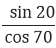Since sin (90 - θ) = cos θTherefore,(ii) Given that,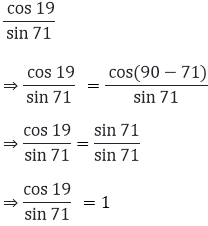Since cos (90 - θ) = sin θ

Therefore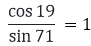(iii) Given that,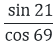Since (90 - θ) = cos θ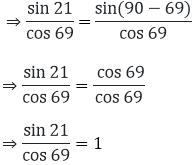(iv) We are given that,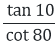Since tan (90 - θ) = cot θTherefore(v) Given that,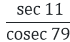Since sec (90 - θ) = cosec θ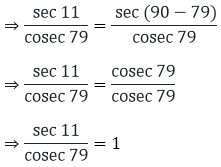Therefore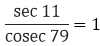### Question: 2

Evaluate the following: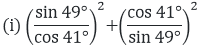(ii) cos 48°- sin 42°(vii) cosec 31° - sec 59°.

(viii) (sin 72° + cos 18°) (sin 72° - cos 18°)

(ix) sin 35° sin 55° - cos 35° cos 55°

(x) tan 48° tan 23° tan 42° tan 67°

(xi) sec 50° sin 40° + cos 40° cosec 50°

### Solution:

(i) We have to find:sin(90° - θ) = cos θ and cos(90°- θ) = sin θ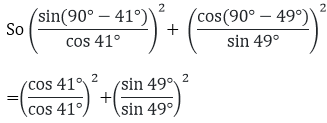= 1 + 1

= 2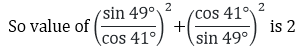(ii) We have to find: cos 48° - sin 42°

Since cos (90° − θ) = sin θ.

So cos 48° - sin 42° = cos (90° − 42°) – sin 42° = sin 42° - sin 42°= 0

So value of cos 48° - sin 42° is 0

(iii) We have to find:Since cot (90°- θ) = tan θ and cos (90°- θ) = sin θ(iv) We have to find: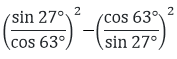Since sin(90°- θ) = cos θ and cos (90 - θ)= 1 - 1

= 0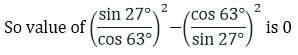(v) We have to find: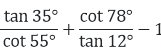Since tan (90°- θ) = cot θ and cot (90°- θ) = tan θ

= 1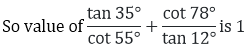(vi) We have to find: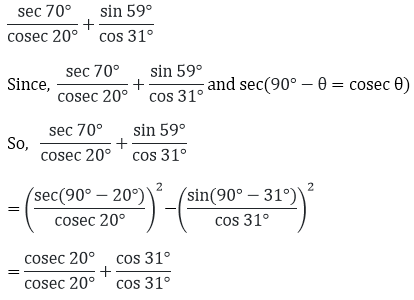= 1 + 1 = 2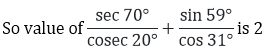(vii) We have to find:

cosec 31° − sec 59°

Since cosec (90° − θ) = sec θ.

So = cosec 31° − sec 59°

= cosec (90° − 59°) – sec 59° = sec 59° − sec 59° = 0

So value of cosec 31° − sec 59° is 0

(viii) We have to find:

(sin 72° + cos 18°)(sin 72° − cos 18°)

Since sin(90° − θ) = cos θ

So (sin 72° + cos 18°) (sin 72° − cos 18°)

= (sin 72°)2 - (cos 18°)2 = [sin(90° − 18°)]2 − (cos18°)2

= (cos18°)2 - (cos18°)2

= cos218°−cos218° = 0

So value of (sin 72° + cos 18°) (sin 72° − cos 18°) is 0.

(ix) We find:

sin 35°sin 55° - cos 35°cos 55°

Since sin(90° − θ) = cos θ and cos(90° − θ) = sin θ sin 35°sin 55°-  cos 35°cos 55°

= sin (90° − 55°) sin 55° - cos(90° − 55°) cos 55°

= 1 - 1

= 0

So value of sin 35° sin 55° - cos 35° cos 55° is 0

(x) We have to find:

tan 48° tan 23° tan 42° tan 67°

Since tan(90°− θ) = cot θ. So tan 48° tan 23° tan 42° tan 67°

= tan (90° − 42°) tan (90° − 67°) tan 42° tan67°

= cot 42°cot 67° tan 42° tan 67°

= (tan 67°cot 67°) (tan 42°cot 42°)

= 1 x 1 = 1

So value of tan 48° tan 23° tan 42° tan 67° is 1

(xi) We have to find:

sec 50° sin 40° + cos 40°cosec 50°

Since cos (90° − θ) = sin θ, sec (90°- θ) = cosec θ and sin θ. cosec θ = 1.

So sec 50° sin 40° + cos 40° cosec 50°

= sec (90° − 40°) sin 40° + cos (90° − 50°) cosec 50°

=1 + 1

= 2

So value of sec 50°sin 40° + cos 40°cosec 50° is 2.

### Question: 3

Express cos 75°+ cot 75° in terms of angle between 0° and 30°

### Solution:

Given that: cos 75° + cot 75°

= cos 75° + cot 75° = cos (90° − 15°) + cot (90° − 15°)

= sin 15° + tan 15°

Hence the correct answer is sin 15° + tan 15°

### Question: 4

If sin 3A = cos (A - 26°), where 3A is an acute angle, find the value of A.

### Solution:

We are given 3A is an acute angle. We have: sin 3A = cos (A - 26°)

⇒ sin 3A = sin(90° - (A – 26°))

⇒ sin3A = sin(116° – A)

⇒ 3A =116° – A

⇒ 4A = 116°

⇒ A = 29°

Hence the correct answer is 29°

### Question: 5

If A, B, C are the interior angles of a triangle ABC, prove that,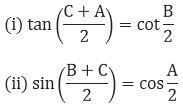### Solution:

(i)  We have to prove: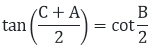Since we know that in triangle ABC

A + B + C = 180

⇒ C + A = 180° – B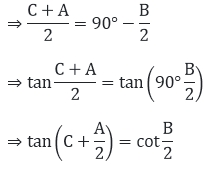Hence proved

(ii) We have to prove: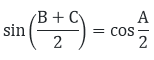Since we know that in triangle ABC

A + B + C = 180

⇒ B + C = 180° – A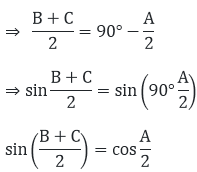Hence proved

### Question: 6

Prove that:

(i) tan 20° tan 35° tan 45° tan 55° tan 70° = 1

(ii) sin 48°. Sec 48° + cos 48°.cosec 42° = 2### Solution:

(i) Therefore tan 20° tan 35° tan 45° tan 55° tan 70°

= tan (90° − 70°) tan (90° − 55°) tan 45°tan 55° tan70°

= cot 70°cot 55° tan 45° tan 55° tan 70°

= (tan 70°cot 70°)(tan 55°cot 55°) tan 45°

= 1 × 1 × 1 = 1

Hence proved

(ii) We will simplify the left hand side

Sin 48°. Sec 48° + cos 48°. Cosec 42°

= sin 48°. sec (90° − 48°) cos 48°. cosec (90° − 48°)

= sin 48°.cos 48° + cos 48°.sin 48°

= 1 + 1 = 2

Hence proved

(iii) We have,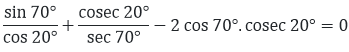So we will calculate left hand side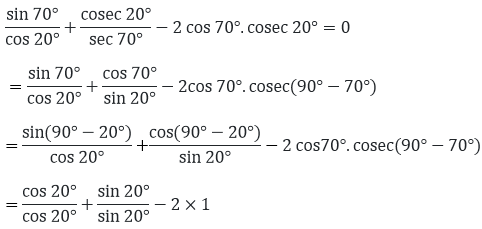= 1 + 1 - 2

= 2 - 2

= 0

Hence proved

(iv) We have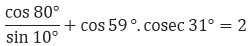We will simplify the left hand side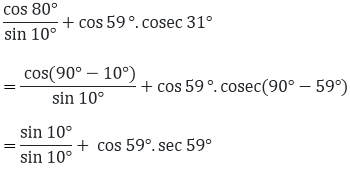= 1 + 1

= 2

Hence proved

### Question: 7

If A, B, C are the interior of triangle ABC, show that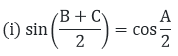### Solution:

(i) A + B + C = 180°

B + C = 180° - A/2

LHS = RHS

### Question: 8

If 2θ + 45° and 30 − θ are acute angles, find the degree measure of θ satisfying sin (20 + 45°) = cos (30° + θ)

### Solution:

Here 2θ + 45° = sin (60° + θ)

We know that, ((90° − θ) = cos (θ)

= sin (2θ + 45°) = sin (90° − (30° - θ))

= sin (2θ + 45°) = sin (90° − 30° - θ)

= sin (2θ + 45°)

= sin (60° + θ)

On equating sin of angle of we get,

= 2θ + 45° = 60° + θ

= θ = 60° - 45°

= θ = 15°

### Question: 9

If θ is appositive acute angle such that sec θ = cosec 60°, find 2cos2θ − 1

### Solution:

We know that,

sec (90° − θ) = cosec2θ = sec θ

= sec (90° − 60°)

= θ = 30°

= 2cos2 θ − 1

= 2cos2 30 − 1### Question: 10

If sin 3θ = cos (θ − 6°) where 3θ and θ – 6° acute angles, find the value of θ.

### Solution:

We have, sin 3θ = cos (θ - 6°) cos (90° + 3θ)

= cos (θ − 6°)

= 90° − 3θ = θ − 6°

− 3θ – θ = - 6° − 90°

− 4θ = – 96°

θ = – 96°/- 4

θ = 24°

### Question: 11

If sec 2A = cosec (A − 42°) where 2A is acute angle, find the value of A.

### Solution:

We know that sec (90 – 3θ) = cosec θ

sec 2A = sec (90 − (A − 42))

sec 2A = sec (90 − A + 42))

sec 2A = sec (132 − A)

Now equating both the angles we get

2A = 132 – A

3A = 132

A = 132/3

A = 44